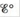# The equation Δ G° = − n F also can be applied to half-reactions. Use standard reduction potentials to estimate Δ G f ∘ for Fe 2+ ( aq ) and Fe 3+ (aq). Δ G f ∘ for e − = 0.)### Chemistry: An Atoms First Approach

2nd Edition
Steven S. Zumdahl + 1 other
Publisher: Cengage Learning
ISBN: 9781305079243

#### Solutions

Chapter
Section### Chemistry: An Atoms First Approach

2nd Edition
Steven S. Zumdahl + 1 other
Publisher: Cengage Learning
ISBN: 9781305079243
Chapter 17, Problem 54E
Textbook Problem
1 views

## The equation ΔG° = −nFalso can be applied to half-reactions. Use standard reduction potentials to estimate Δ G f ∘ for Fe2+ (aq) and Fe3+ (aq). Δ G f ∘ for e − = 0.)

Interpretation Introduction

Interpretation:

The value of ΔG°f for Fe2+(aq) and Fe3+(aq) using their standard reduction values is to calculated.

Concept introduction:

The species with higher reduction potential undergoes reduction at the cathode while the species with lower reduction potential undergoes oxidation at the anode. The change in Gibbs free energy is the useful amount work that can be done and negative value of ΔG° shows that the reaction is spontaneous in nature.

To determine: The value of ΔG°f for Fe2+(aq) and Fe3+(aq) using their standard reduction values.

### Explanation of Solution

The value of ΔG°f(Fe2+(aq)) is calculated as,

ΔG°f(Fe2+(aq))=-84.90kJ_

The value of ΔG°f(Fe3+(aq)) is calculated as,

ΔG°f(Fe2+(aq))=-10.42kJ_

The reduction half-reaction for Fe2+ is represented as follows,

Fe2+(aq)+2eFe(s)E°=0.44V

The value of change in Gibbs free energy for the species involved in the reactions is as follows,

ΔG°f(Fe(s))=0ΔG°f(e)=0

The value of ΔG° for the half-reaction is given by the formula,

ΔG°=nFE°cell

Where,

• ΔG° is the Gibbs free energy change at the standard conditions.
• n is the number of electrons involved in the reaction.
• F is the Faraday’s constant.
• E°cell is the cell potential at the standard condition.

The number of moles of electron involved in the reaction is 2 .

Substitute the values in above equation as,

ΔG°=(2mole)(96,485Cmole)(0.44V)=84,906.8J=84.90kJ

The value of ΔG° is calculated using the formula,

ΔG°=npΔG°f(products)nrΔG°f(reactants)

Where,

• ΔG° is the Gibbs free energy change for the entire cell.
• ΔG°f(products) is the Gibbs free energy change for the products.
• ΔG°f(reactants) is the Gibbs free energy change for the reactants.
• np is the total number of moles of the products.
• nr is the total number of moles of the reactants.

Substitute the values in above equation to calculate the value of ΔG°f(Fe2+(aq)) ,

ΔG°=ΔG°f(Fe(s))(ΔG°f(Fe2+(aq))+ΔG°f(e))42.453kJ=0(ΔG°f(Fe2+(aq))+0)

The value of ΔG°f(Fe2+(aq)) is calculated as,

ΔG°f(Fe2+(aq))=-84

### Still sussing out bartleby?

Check out a sample textbook solution.

See a sample solution

#### The Solution to Your Study Problems

Bartleby provides explanations to thousands of textbook problems written by our experts, many with advanced degrees!

Get Started

Find more solutions based on key concepts
A benefit to health is seen when is used in place of in the diet. a. saturated fat/mono unsaturated fat b. satu...

Nutrition: Concepts and Controversies - Standalone book (MindTap Course List)

What is hereditarianism, and what is the invalid assumption it makes?

Human Heredity: Principles and Issues (MindTap Course List)

(a) Two spheres have radii a and b, and their centers are a distance d apart. Show that the capacitance of this...

Physics for Scientists and Engineers, Technology Update (No access codes included)